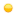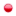###Author Topic: What's the optimal number of threads in parallel computations?  (Read 1695 times)

#### chrisapo

• Newbie
•• Posts: 4##### What's the optimal number of threads in parallel computations?
Hello.
Regarding hybridly parallel computations, here's an excerpt from MCTDH-X's manual:
Quote
the most efficient way is usually to run MCTDH-X with at least as many MPI processes as there are orbitals. The OpenMP shared memory parallelization takes care of efficiently performing the computational task inside of each MPI process."

So, it is suggested a rule of thumb for the number of MPI processes: at least as many as there are orbitals. My question is then: is there a similar rule of thumb for the appropriate number of OpenMP threads (per MPI process)?

#### mctdhb

• Newbie
•• Posts: 15##### Re: What's the optimal number of threads in parallel computations?
Hi Chrisapo,

generally, the parallel performance is computation- and environment dependent. You will just have to test out what works best.

Judging from your previously posted examples, I checked the following:
For a 2D example with 128x128 grid points, N=100 particles and M=4 orbitals, I get reasonable performance using just OpenMP threads with a single MPI process on my desktop computer. In that case, using more than 4 threads didn't add much performance.

Hope that helps!